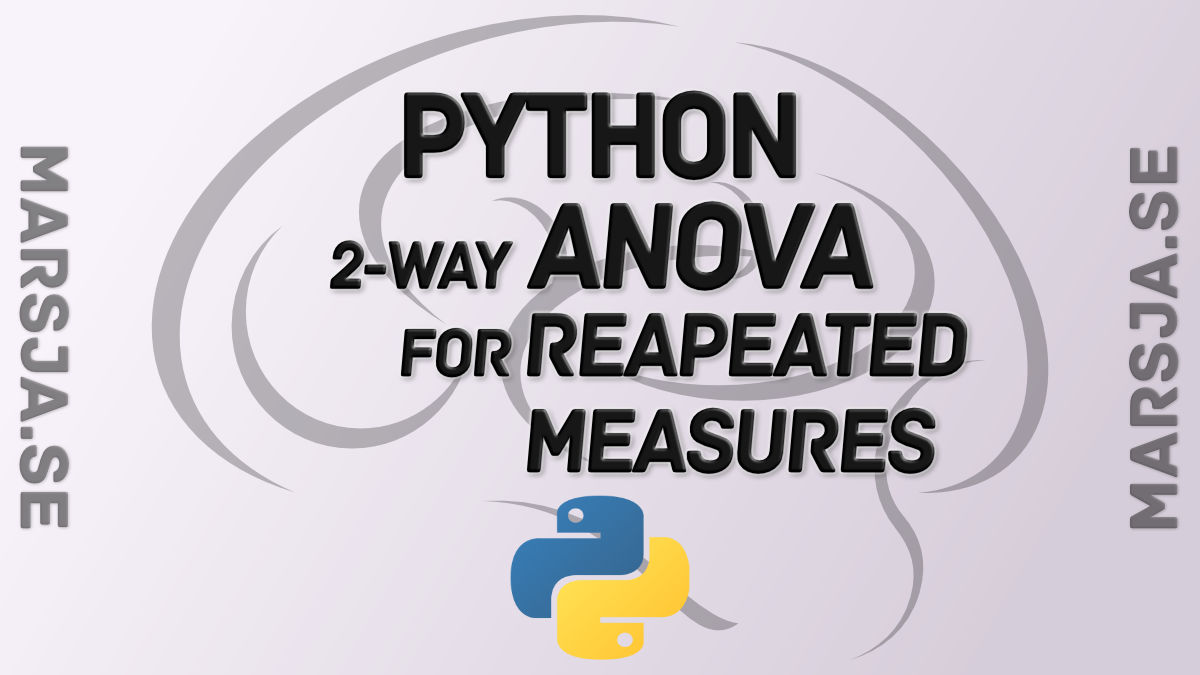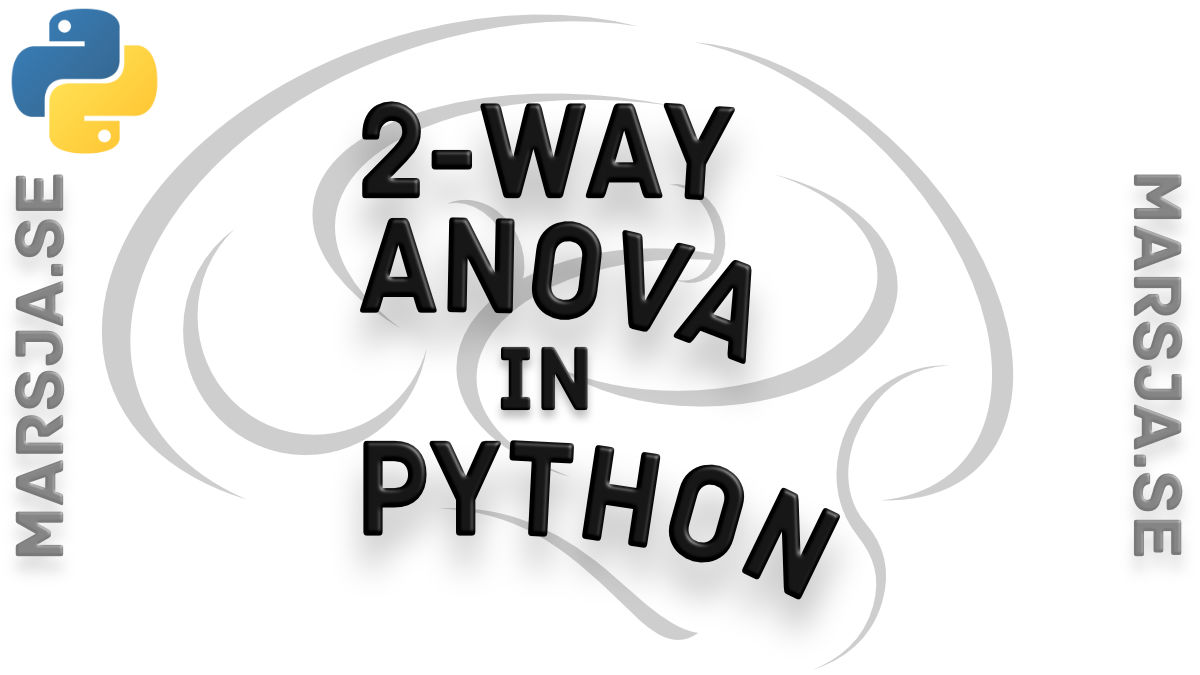## Two-way ANOVA for repeated measures using Python

Previously I have shown how to analyze data collected using within-subjects designs using rpy2 (i.e., R from within Python) and Pyvttbl. In this post I will extend it into a factorial ANOVA using Python (i.e., Pyvttbl). In fact, we are going to carry out a Two-way...## Three ways to do a two-way ANOVA with Python

In an earlier post, I showed four different techniques that enable a one-way analysis of variance (ANOVA) using Python. Now, in this post, we, are going to learn how to do two-way ANOVA for independent measures using Python. First, we are going to learn how to...